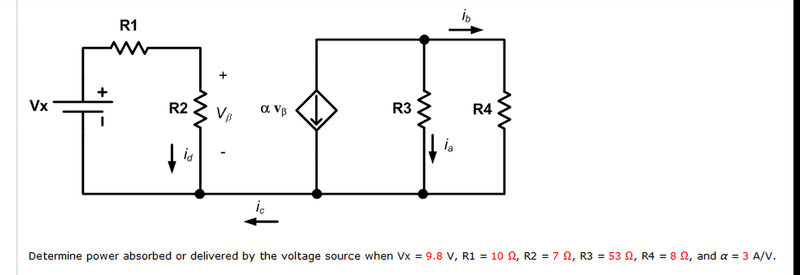# Find power absorbed or delivered

Cisneros778

## Homework StatementKVL

## The Attempt at a Solution

I am confused about what happens at the first loop on the left. It looks like there is a current of i(d) leaving R2 which means that it's the same current for R1 and Vx. But then there is i(c) and I cannot understand what happens to this loop now.
When looking at this, the only way I can make sense of this is to have i(d)=i(c), but even this doesn't make much sense to me because it wouldn't pass the nodal analysis.

Last edited:

## Answers and Replies

voko
What is the thing denoted by ## \alpha \mathbf{v}_{\beta} ##? I do not recognize the symbol.

Homework Helper
Gold Member
That thing is a dependant current source. Regarding id and ic, KCL should tell you what ic is.

Cisneros778
Sorry, that is what I meant when I said it didn't satisfy my nodal analysis.
For KCL- I have:

ic + id + ix =0

but ix is id isn't it?

so is ic + id + id =0
ic = -2id
???

Homework Helper
Gold Member
For KCL- I have:

ic + id + ix =0

but ix is id isn't it?

Yes it is. Watch your sign. It is going into and outfrom that node.

Mentor
Take note that the wire denoted as carrying ##i_c## forms a single path between the loops; there is no return path, so no complete circuit exists for ##i_c## to flow. What does this imply about the value of ##i_c##?

Cisneros778
so i(c) is zero.

if i(d) is going in and going out.

i(d) - i(d) + i(c) = 0
i(c) = 0

so now that I know that:
-9.8 + 10*i(d) + 7*i(d) = 0
17*i(d) = 9.8
i(d) = .576 A

Absorbed power is=
(.576 A)^2 * R2 = 2.33 W

which is wrong, the correct answer is -0.00851 W... So i'm stuck again.

Mentor
Something must be fishy with the question then. The loop with Vx in it is effectively all alone, so the current is 0.576A as you calculated. Since Vx is 9V, the power it's producing must be V*I = 5.65W. (Note that BOTH resistors will absorb power, not just R2).

Is it possible that some of the component values stated in the image are incorrect?

Cisneros778
I don't think so. The reason I used P = i^2*R2 is because of that V(b).. I figured the question was referring to that or else why would it be there? What do you think?

Mentor
##V_\beta## is there because the voltage across R2 is used to set the current of the controlled source in the second loop. The only voltage source Vx.